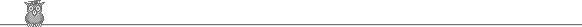### IMO 1981Problem A3

Determine the maximum value of m2 + n2, where m and n are integers in the range 1, 2, ... , 1981 satisfying (n2 - mn - m2)2 = 1.

Solution

Experimenting with small values suggests that the solutions of n2 - mn - m2 = 1 or -1 are successive Fibonacci numbers. So suppose n > m is a solution. This suggests trying m+n, n: (m+n)2 - (m+n)n - n2 = m2 + mn - n2 = -(n2 - mn - m2) = 1 or -1. So if n > m is a solution, then m+n, n is another solution. Running this forward from 2,1 gives 3,2; 5,3; 8,5; 13,8; 21,13; 34,21; 55,34; 89,55; 144,89; 233,144; 377,233; 610,377; 987,610; 1597,987; 2584,1597.

But how do we know that there are no other solutions? The trick is to run the recurrence the other way. For suppose n > m is a solution, then try m, n-m: m2 - m(n-m) - (n-m)2 = m2 + mn - n2 = -(n2 - mn - m2) = 1 or -1, so that also satisfies the equation. Also if m > 1, then m > n-m (for if not, then n >= 2m, so n(n - m) >= 2m2, so n2 - nm - m2 >= m2 > 1). So given a solution n > m with m > 1, we have a smaller solution m > n-m. This process must eventually terminate, so it must finish at a solution n, 1 with n > 1. But the only such solution is 2, 1. Hence the starting solution must have been in the forward sequence from 2, 1.

Hence the solution to the problem stated is 15972 + 9872.Solutions are also available in     Murray S Klamkin, International Mathematical Olympiads 1978-1985, MAA 1986, and in   István Reiman, International Mathematical Olympiad 1959-1999, ISBN 189-8855-48-X.